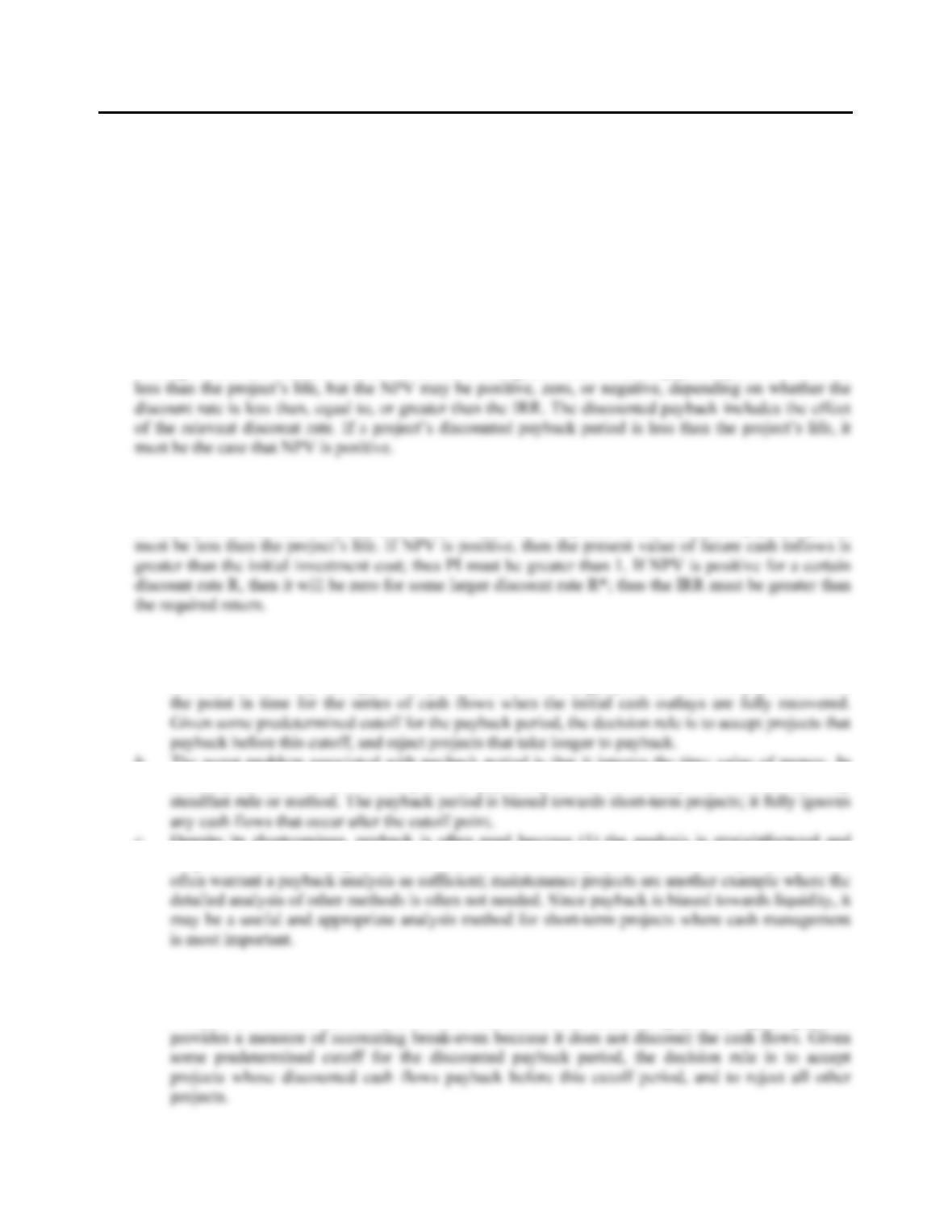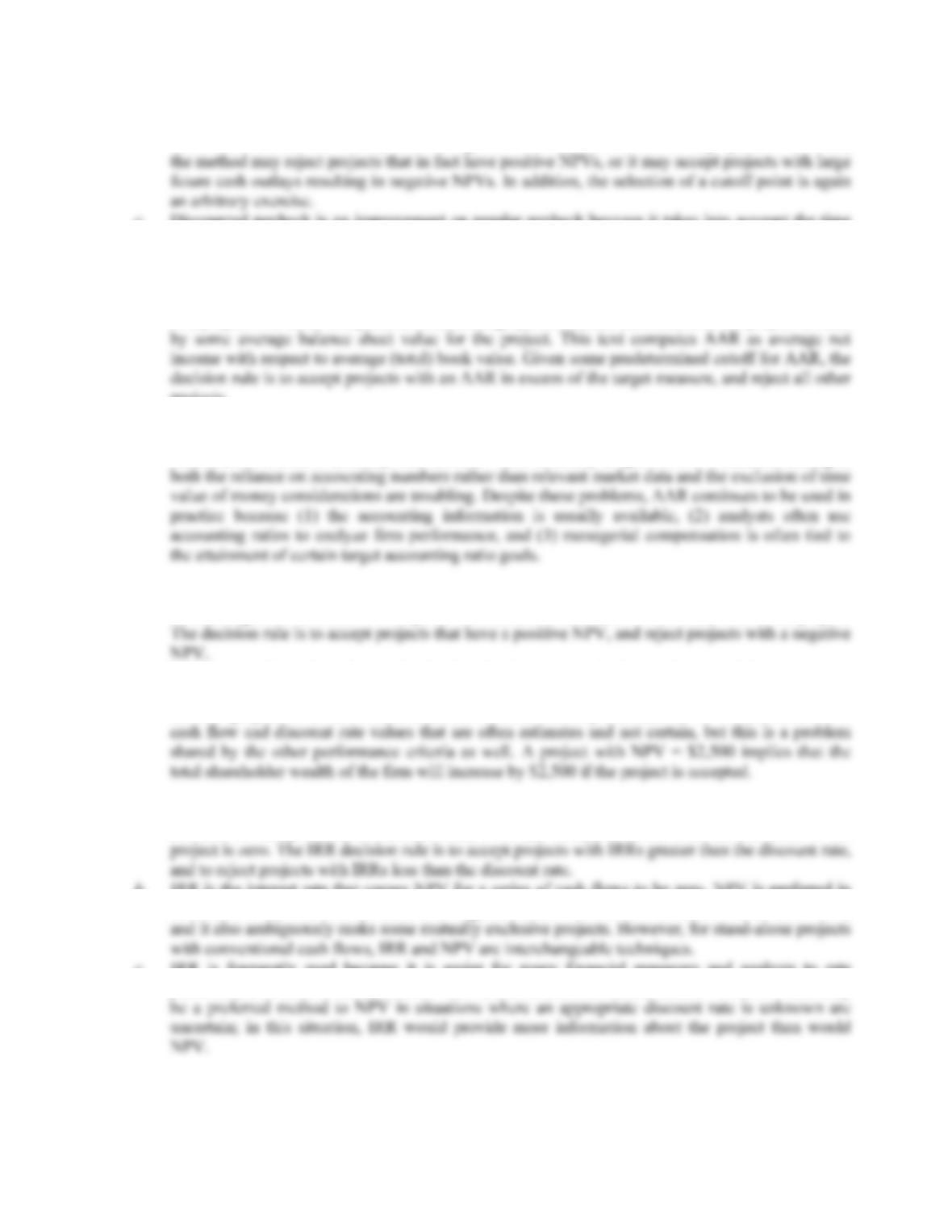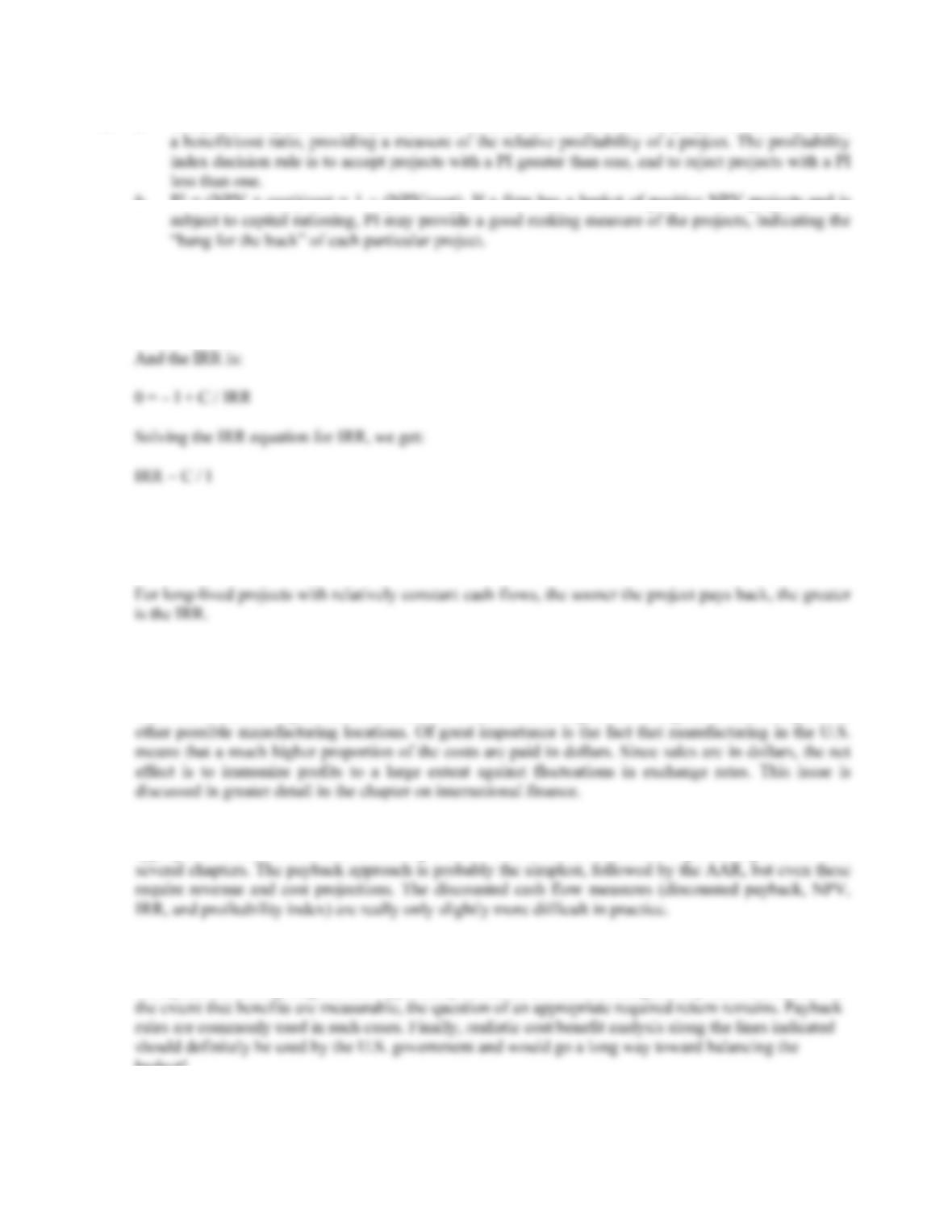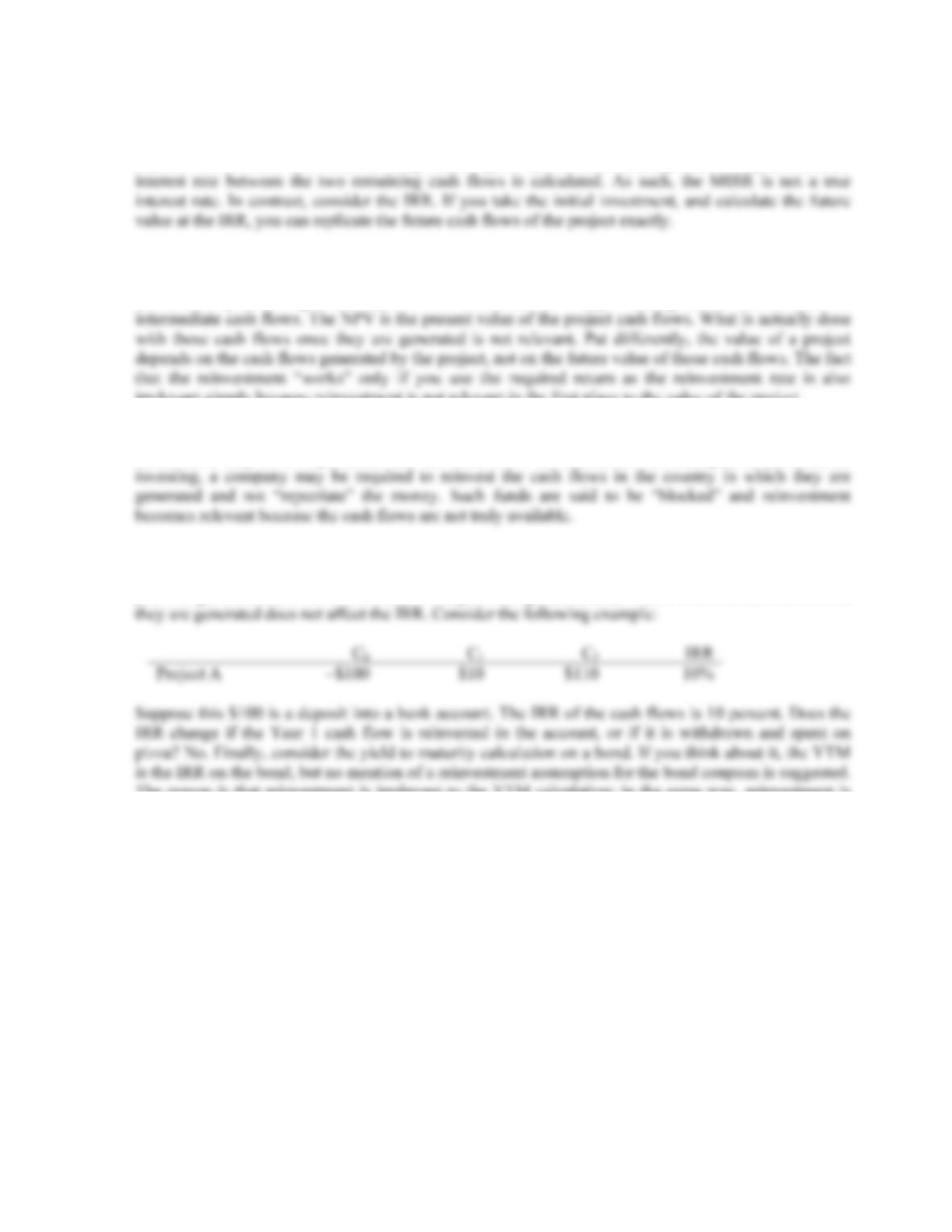# 978-0073382395 Chapter 9 Concepts Review and Critical Thinking Questions

Document Type
Homework Help
Book Title
Fundamentals of Corporate Finance Standard Edition 9th Edition
Authors
Stephen RossCHAPTER 9
NET PRESENT VALUE AND OTHER
INVESTMENT CRITERIA
Answers to Concepts Review and Critical Thinking Questions
1. A payback period less than the project’s life means that the NPV is positive for a zero discount rate, but
nothing more definitive can be said. For discount rates greater than zero, the payback period will still be
2. If a project has a positive NPV for a certain discount rate, then it will also have a positive NPV for a
zero discount rate; thus, the payback period must be less than the project life. Since discounted payback
is calculated at the same discount rate as is NPV, if NPV is positive, the discounted payback period
3. a. Payback period is simply the accounting break-even point of a series of cash flows. To actually
compute the payback period, it is assumed that any cash flow occurring during a given period is
realized continuously throughout the period, and not at a single point in time. The payback is then
b. The worst problem associated with payback period is that it ignores the time value of money. In
addition, the selection of a hurdle point for payback period is an arbitrary exercise that lacks any
c. Despite its shortcomings, payback is often used because (1) the analysis is straightforward and
simple and (2) accounting numbers and estimates are readily available. Materiality considerations
4. a. The discounted payback is calculated the same as is regular payback, with the exception that each
cash flow in the series is first converted to its present value. Thus discounted payback provides a
measure of financial/economic break-even because of this discounting, just as regular paybackB-154 SOLUTIONS
b. The primary disadvantage to using the discounted payback method is that it ignores all cash flows
that occur after the cutoff date, thus biasing this criterion towards short-term projects. As a result,
c. Discounted payback is an improvement on regular payback because it takes into account the time
value of money. For conventional cash flows and strictly positive discount rates, the discounted
payback will always be greater than the regular payback period.
5. a. The average accounting return is interpreted as an average measure of the accounting performance
of a project over time, computed as some average profit measure attributable to the project divided
projects.
b. AAR is not a measure of cash flows and market value, but a measure of financial statement
accounts that often bear little resemblance to the relevant value of a project. In addition, the
selection of a cutoff is arbitrary, and the time value of money is ignored. For a financial manager,
6. a. NPV is simply the present value of a project’s cash flows. NPV specifically measures, after
considering the time value of money, the net increase or decrease in firm wealth due to the project.
NPV.
b. NPV is superior to the other methods of analysis presented in the text because it has no serious
flaws. The method unambiguously ranks mutually exclusive projects, and can differentiate
between projects of different scale and time horizon. The only drawback to NPV is that it relies on
7. a. The IRR is the discount rate that causes the NPV of a series of cash flows to be exactly zero. IRR
can thus be interpreted as a financial break-even rate of return; at the IRR, the net value of the
b. IRR is the interest rate that causes NPV for a series of cash flows to be zero. NPV is preferred in
all situations to IRR; IRR can lead to ambiguous results if there are non-conventional cash flows,
c. IRR is frequently used because it is easier for many financial managers and analysts to rate
performance in relative terms, such as “12%”, than in absolute terms, such as “\$46,000.” IRR mayCHAPTER 9 B-155
8. a. The profitability index is the present value of cash inflows relative to the project cost. As such, it is
b. PI = (NPV + cost)/cost = 1 + (NPV/cost). If a firm has a basket of positive NPV projects and is
9. For a project with future cash flows that are an annuity:
Payback = I / C
Notice this is just the reciprocal of the payback. So:
IRR = 1 / PB
10. There are a number of reasons. Two of the most important have to do with transportation costs and
exchange rates. Manufacturing in the U.S. places the finished product much closer to the point of sale,
resulting in significant savings in transportation costs. It also reduces inventories because goods spend
less time in transit. Higher labor costs tend to offset these savings to some degree, at least compared to
11. The single biggest difficulty, by far, is coming up with reliable cash flow estimates. Determining an
appropriate discount rate is also not a simple task. These issues are discussed in greater depth in the next
12. Yes, they are. Such entities generally need to allocate available capital efficiently, just as for-profits do.
However, it is frequently the case that the “revenues” from not-for-profit ventures are not tangible. For
example, charitable giving has real opportunity costs, but the benefits are generally hard to measure. To
budget!B-156 SOLUTIONS
13. The MIRR is calculated by finding the present value of all cash outflows, the future value of all cash
inflows to the end of the project, and then calculating the IRR of the two cash flows. As a result, the
cash flows have been discounted or compounded by one interest rate (the required return), and then the
14. The statement is incorrect. It is true that if you calculate the future value of all intermediate cash flows
to the end of the project at the required return, then calculate the NPV of this future value and the initial
investment, you will get the same NPV. However, NPV says nothing about reinvestment of
irrelevant simply because reinvestment is not relevant in the first place to the value of the project.
One caveat: Our discussion here assumes that the cash flows are truly available once they are
generated, meaning that it is up to firm management to decide what to do with the cash flows. In certain
cases, there may be a requirement that the cash flows be reinvested. For example, in international
15. The statement is incorrect. It is true that if you calculate the future value of all intermediate cash flows
to the end of the project at the IRR, then calculate the IRR of this future value and the initial investment,
you will get the same IRR. However, as in the previous question, what is done with the cash flows once
The reason is that reinvestment is irrelevant to the YTM calculation; in the same way, reinvestment is
irrelevant in the IRR calculation. Our caveat about blocked funds applies here as well.

## Trusted by Thousands ofStudents

Here are what students say about us.